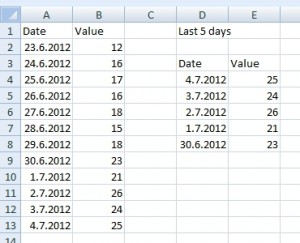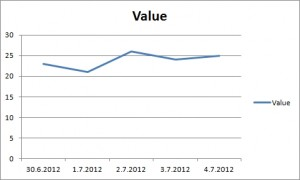# Automatic change in the chart according to the current date

Let’s have a list of some values. The values are taken every day. The task is to create chart showing only last 5 days from today. Charts must be updated automatically.

Important: I wrote this on 4.7.2012, so for this example is 4.7.2012 the current date.

## Solution

### Step 1 / new table

We create a new table that shows only last 5 days from today.First of all we have to determine current date. Formula in D4 is:

=TODAY()

Then we subtract 1, 2, 3 and 4 days from the current day. Formula in D5 is:

=D4-1

Now, we use VLOOKUP function to find right values to the dates. Formula in E4 is:

=VLOOKUP(D4,A:B,2,0)Argument “Table array” is A:B because we need include all values in column A and B. Therefore, we can add more values to the list of dates and this formula will work correctly.

### Step 2 / chart

We create new chart from this auxiliary table. I choose Line chart, but it’s up to you which one you need.1.